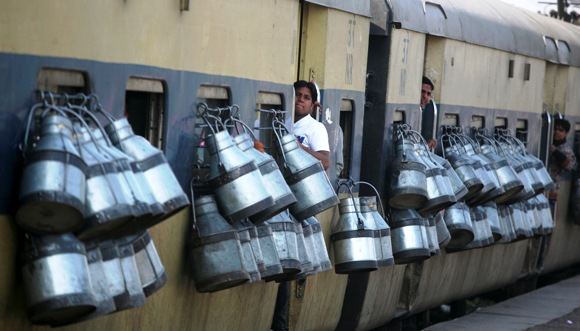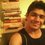# Milk Containers Puzzle - Hardest Logic Puzzle Problem I ever faced.In India, everyday the morning train is overloaded with a lot of milk cans hanged on the windows as you can see above. The five persons named BHEEM, KABIR, TULSI, KAALI, and ZAKIR, who were great planners, planned to steal milk from the bypassing train.So one of them was already in the train. He, then, pulled the chain to stop the train and the other four were already standing at the place of event. The train stopped and all the five ran away by stealing one can each. Finally, they escaped and reached at the safe place. What they found is that they stole $3$ cans of $32 L$ each, one can of $24 L$ and one can of $7 L$. They got disappointed when they found that only the can of $32 L$ each are full of milk, while that of $24 L$ and $7 L$ are totally empty.

So they decided to divide the milk into the five. The two, who brought the empty cans with them, will get smaller amount of milk than the rest three.So $3$ persons, who stole cans with milk, will get $24 L$ each, while the two, who stole empty cans, will get $24 L$ together (12 L each). For that, they decided to equally divided the milk into the four cans (three cans of $32 L$ and one can of $24L$).

There are five containers of milk, lets name them : $\color{#20A900}{A \rightarrow \text{Container with 32 L capacity} \\ B \rightarrow \text{Container wit 32 L capacity} \\ C \rightarrow \text{Container with 32 L capacity} \\ D \rightarrow \text{Container with 24 L capacity} \\ E \rightarrow \text{Container with 7 L capacity}}$

Initial Situation : The containers $A,B,C$ are having $32 L$ of milk in each of them, and the containers $D$ and $E$ are empty.

Final Situation to be reached : Container$A$, container $B$, container $C$ and container $D$ are having $24 L$ of milk each and container $E$ is empty.

Conditions : (How to reach the Final Situation)

1. You have to transfer the milk from one container to the other(s) to achieve the Final Situation.

2. You can only use the given containers i.e. Container $A$, container $B$ , container $C$, container $D$ and container $E$ for the purpose of measuring and transferring.

3. Obviously, you aren't supposed to use the approximations of the milk and the containers. So, the measure must be exact.

4. You're supposed to be perfect in the job of measuring and transferring milk (:P). So you don't let even a single drop of milk wasted in the whole process while transferring the milk from one container to the other(s).

What steps you will take to reach the Final Situation ?

Please post your solution step by step.

enjoy

$\Large \text{Thanks !}$Note by Sandeep Bhardwaj
6 years ago

This discussion board is a place to discuss our Daily Challenges and the math and science related to those challenges. Explanations are more than just a solution — they should explain the steps and thinking strategies that you used to obtain the solution. Comments should further the discussion of math and science.

When posting on Brilliant:

• Use the emojis to react to an explanation, whether you're congratulating a job well done , or just really confused .
• Ask specific questions about the challenge or the steps in somebody's explanation. Well-posed questions can add a lot to the discussion, but posting "I don't understand!" doesn't help anyone.
• Try to contribute something new to the discussion, whether it is an extension, generalization or other idea related to the challenge.
• Stay on topic — we're all here to learn more about math and science, not to hear about your favorite get-rich-quick scheme or current world events.

MarkdownAppears as
*italics* or _italics_ italics
**bold** or __bold__ bold
- bulleted- list
• bulleted
• list
1. numbered2. list
1. numbered
2. list
Note: you must add a full line of space before and after lists for them to show up correctly
paragraph 1paragraph 2

paragraph 1

paragraph 2

[example link](https://brilliant.org)example link
> This is a quote
This is a quote
    # I indented these lines
# 4 spaces, and now they show
# up as a code block.

print "hello world"
# I indented these lines
# 4 spaces, and now they show
# up as a code block.

print "hello world"
MathAppears as
Remember to wrap math in $$ ... $$ or $ ... $ to ensure proper formatting.
2 \times 3 $2 \times 3$
2^{34} $2^{34}$
a_{i-1} $a_{i-1}$
\frac{2}{3} $\frac{2}{3}$
\sqrt{2} $\sqrt{2}$
\sum_{i=1}^3 $\sum_{i=1}^3$
\sin \theta $\sin \theta$
\boxed{123} $\boxed{123}$

## Comments

Sort by:

Top Newest

A generic approach for solving such problems would be to first define a directed graph $\mathcal{G}(V,E)$ with $V$ being the set of all $5$-tuples $(a_1,a_2,a_3,a_4,a_5), 0\leq a_i \leq C_i, a_i \in \mathbb{Z}_+$, with $C_i$ being the capacities of the containers (here $32,32,32,24,7$ respectively). Then from each vertex $v\in V$ we define at most $2\times\binom{5}{2}=20$ directed edges, each one pointing to a vertex corresponding to selecting two containers in $v$ and transferring the content from the first to the second until the first one becomes empty or the second one becomes full. The definition of $\mathcal{G}$ is now complete. Now the problem becomes finding a shortest path from the initial situation to the final situation (if there is any).

- 6 years ago

Log in to reply

So can you find the shortest path using graph theory ?

- 6 years ago

Log in to reply

Yes, e.g., by a Breadth First Search.

- 6 years ago

Log in to reply

Sir I also have a logical problem which is as follows

3 friends went to eat burger in McDonalds.They had a total combined 75rs. The cost of each burger is 25rs but as they were happy hour customer they got a discount of 5rs.So they gave 2rs tip to the waiter and distributed the remaining 3rs among them.Money spent by each of them-(25-1)=24rs. They calculated how much money they had spent which is as follows

Money spent by each person=24rs*3

Tip given=2rs

Total comes out to be 24*3=72+2=74

But they had brought 75rs.So where did 1rs go???

P.S Don't try to find out grammatical mistakes..

- 6 years ago

Log in to reply

75 Rs =70 Rs ( McDonal expense) + 2 Rs (tip to the waiter) + 3 Rs (back to 3 friends)

You're trying to troll the audience by adding the different quantities.

- 6 years ago

Log in to reply

How r they different quantities I have just added the total expense by each person and their mutual expense

- 6 years ago

Log in to reply

Total Money = Money spent + Money left

$\implies$ Rs 75 ( total money) = Rs 72 ( Money spent : 70 - McDonald account, 2- tip to the waiter) + Rs 3 (Money left i.e. the money returned to them)

I mean to say different quantities is that you're trying to say : Total money=Money spent by them + Money in account's waiter. But there is no relation of money spent by them and the money in the waiter's account. The tip to the waiter is just a part of their money spent.

- 6 years ago

Log in to reply

×

Problem Loading...

Note Loading...

Set Loading...